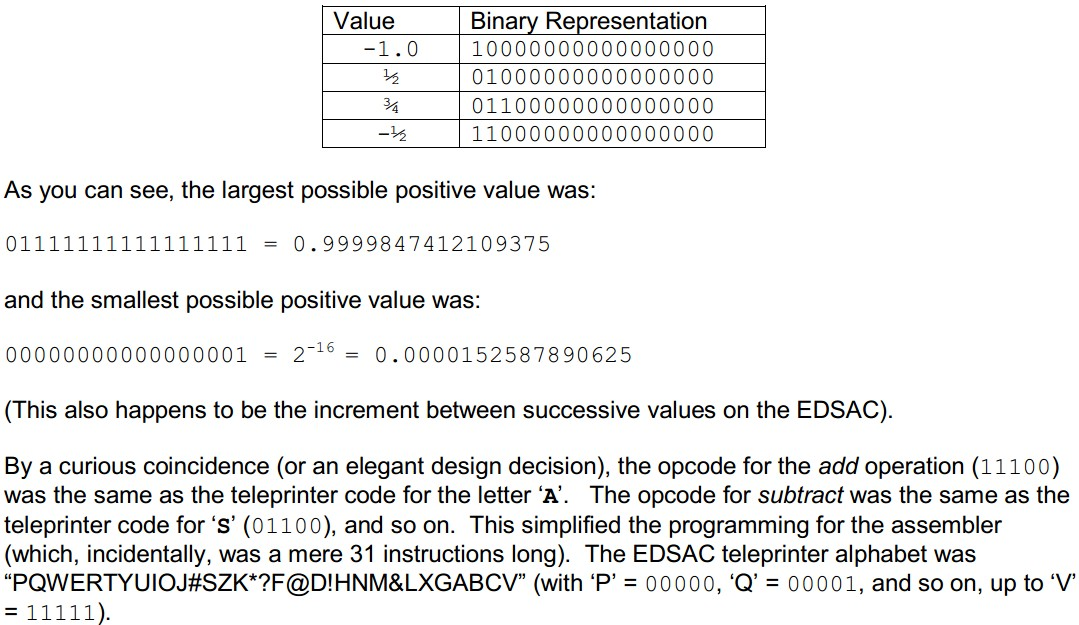﻿ N-B-U-T Online Judge ::  Programming the EDSAC
• ###  Programming the EDSAC

• 时间限制: 1000 ms　内存限制: 65535 K
• 问题描述
•• 输入
• The first line of input contains a single integer P, (1 <= P <= 1000), which is the number of data sets that follow. Each data set is a single line that consists of two space separated values N and D. N is the data set number. D is the decimal number of the form sd.ddd...., where s is an optional minus sign, and d is any decimal digit (0 - 9). There will be at least 1 and at most 16 digits after the decimal point.
• 输出
• For each data set there is one line of output. It contains the data set number (N) followed by a single space, followed by the EDSAC instruction necessary to specify the given constant. The instruction should be printed as follows: the "opcode" character followed by a space followed by the operand (as a non-negative decimal integer) followed by a space followed by an 'F' or 'D' (as appropriate). If the constant cannot be represented exactly in 17 bits, the value is to be rounded toward zero (up for negative, down for positive numbers). If the input value D is not in the range -1.0 <= D <= 1.0, the string "INVALID VALUE" should be printed instead of an EDSAC instruction.
• 样例输入
• ```16
1 0.5
2 -0.5
3 -1.0000000
4 0.1
5 0.000015258789625
6 0.000015258789624
7 0.000015258789626
8 -0.000015258789625
9 -0.000015258789624
10 -0.000015258789626
11 0.9999999999999999
12 -0.9999999999999999
13 -5.3
14 9.1
15 -1.0000000000000001
16 0.31415926
```
• 样例输出
• ```1 I 0 F
2 & 0 F
3 ? 0 F
4 Q 1228 D
5 P 0 D
6 P 0 F
7 P 0 D
8 V 2047 D
9 P 0 F
10 V 2047 D
11 * 2047 D
12 ? 0 D
13 INVALID VALUE
14 INVALID VALUE
15 INVALID VALUE
16 T 54 F
```
• 提示
• `无`
• 来源
• `Greater New York Regional`
• 操作
﻿